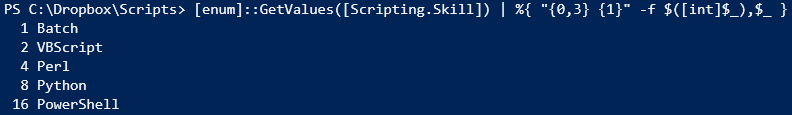# #PSTip Displaying all possible combinations of a flagged enumeration

In the last tip, I showed you how to create an enumeration object on which we can do bitwise operations to derive combinational values. Now, in this tip, let us see how to retrieve all possible combinations.

Assuming that we forgot the values assigned to each enumeration constant, let us first see how to see the values of each constant:

```[Enum]::GetValues([Scripting.Skill]) | % { "{0,3} {1}" -f \$([int]\$_),\$_ }
```

So, this gives us something similar to:Since the total of all these values is 31, let us use how to derive all possible combinations.

```for (\$i = 0; \$i -lt 31; \$i++) {
"{0,3} {1}" -f \$([int]\$i), [Scripting.Skill]\$([int]\$i)
}
```

This output is generally longer! So, try it yourself!

Share on: